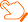### 近期论文查看导师最新文章 （温馨提示：请注意重名现象，建议点开原文通过作者单位确认）

1]（T. Y. Hou, Z. Q. Shi，王术）On singularity formation of a 3D model for incompressible Navier-Stokes equations. Advances in Math. (美国),230(2012), 607-641. SCI （T. Y. Hou, C. M. Li, Z. Q. Shi, 王术, X. W. Yu）On singularity formation of a nonlinear nonlocal system. Arch. Rational Mech. Anal.,199(2011), 117-144. SCI （王术，K. Wang）The mixed layer problem and quasi-neutral limit of the drift-diffusion model for semiconductors, SIAM J Math. Anal.,44(2)(2012),699-717. SCI （王术, Y. H. Feng，X. Li）The asymptotic behavior of globally smooth solutions of bipolar non-isentropic compressible Euler-Maxwell system for plasma, SIAM J Math. Anal., 44(5) (2012), 3429–3457. SCI （Y. J. Peng, 王术）The convergence of Euler-Maxwell system to the incompressible Euler equation，Commun. in Partial Differential Equation, 33(2008)，349-376. SCI  王术编著，Sobolev空间与偏微分方程引论，北京：科学出版社，2009年4月。 （Y. Ueda, 王术，S. Kawashima）Dissipative structure of the regularity-loss type and time asymptotic decay of solutions for the Euler--Maxwell system, SIAM J Math. Anal.,44(3)(2012), 2002-2017. （Y. J. Peng, 王术, Q. L. Gu）Relaxation limit and global existence of smooth solutions of compressible Euler-Maxwell equations，SIAM J Math. Anal.,43(2)(2011), 944-970. SCI （Y. J. Peng, 王术）Rigorous derivation of incompressible e-MHD equations from compressible Euler-Maxwell equations, SIAM J Math. Anal.,40(2)(2008), 540-565. SCI （王术, Z. P. Xin，P. A. Markowich）Quasineutral limit of drift-diffusion models for semiconductors: general doping profile case, SIAM J Math. Anal. 37(6)(2006)，1854-1889. SCI （王术，S. Jiang）The convergence of Navier-Stokes-Poisson system to the incompressible Euler equation，Commun. in Partial Differential Equation, 31(2006), 1-21. SCI （王术）Quasineutral limit of Euler-Poisson system with and without viscosity, Commun. in Partial Differential Equations, 29(3&4)(2004), 419-456. SCI （A. Jüngel，王术）Convergence of nonlinear Schrödinger-Poisson systems to the compressible Euler equations, Commun. in Partial Differential Equations, 28(2003), 1005-1022. SCI （Q. H. Shi，王术，Y. Li）Existence and uniqueness of energy solution to Klein–Gordon –Schrodinger equations, Journal of Differential Equations, 252(2012), 168–180. SCI （K. Wang，王术）Quasi-neutral limit to the drift-diffusion models for semiconductors with physical contact-insulating boundary conditions.Journal of Differential Equations, 249(2010), 3291-3311. SCI （L. Hsiao，王术）Quasineutral limit of a time-dependent drift-diffusion-Poisson models for PN junction semiconductor devices, Journal of Differential Equations, 225（2006）,411-439. SCI （L. Hsiao，P. A. Markowich，王术）Asymptotic behavior of globally smooth solutions to the multidimensional isentropic hydrodynamic model for semiconductors, Journal of Differential Equations, 192(2003), 111-133. SCI （王术）Doubly nonlinear degenerate parabolic systems with coupled nonlinear boundary conditions, Journal of Differential Equations, 182(2002), 431-469. SCI （王术，M. X. Wang，C. H. Xie）Quasi-linear parabolic systems with nonlinear boundary conditions, Journal of Differential Equations, 166(2000), 251-265. SCI （Q. C. Ju,王术）Quasi-neutral limit of the multidimensional drift-diffusion models for semiconductors, Math. Models Methods Appl. Sci. (M3AS), 20(9)（2010），1649-1679. SCI （王术）Quasineutral limit of multi-dimensional drift-diffusion model，Math. Models Methods Appl. Sci.(M3AS), 16(2006)，537-557. SCI （C. Schmeiser，王术）Quasineutral limit of the drift diffusion models for semiconductors with the general initial data, Math. Models and Methods Appl. Sci.(M3AS)，13(4)(2003), 463-470. SCI （L. Hsiao，王术）Asymptotic behavior of global smooth solutions to the Full 1D hydrodynamic model for semiconductors, Math. Models Methods Appl. Sci.(M3AS), 12(2002), 777-796. SCI （Q. H. Shi，王术，Y. Li，C. Y. Wang）Well posedness for the nonlinear Klein-Gordon -Schrodinger equations with hetero-interactions. J. Math. Phys., 51(3)(2010), 032102. SCI （J. W. Yang，王术）Convergence of the non-isentropic Euler-Maxwell equations to compressible Euler-Poisson equations. J. Math. Phys., 50(12)(2009), 123508, 15pp. SCI （Q. C. Ju，Y. Li，王术）Rate of convergence from the Navier-Stokes-Poisson system to the incompressible Euler equations. J. Math. Phys., 50(1)(2009), 013533, 12 pp. SCI （Q. C. Ju, F. C. Li，王术）Convergence of the Navier-Stokes-Poisson system to the incompressible Navier-Stokes equations，J. Math. Phys., 49(7) (2008), 073515, 8pp. SCI

down
wechat